# Ricci-flat metric

This article defines a property that makes sense for a Riemannian metric over a differential manifold

This property of a Riemannian metric is Ricci flow-preserved, that is, it is preserved under the forward Ricci flow

This is the property of the following curvature being everywhere zero: Ricci curvature

## Definition

### Symbol-free definition

A Riemannian metric on a differential manifold is said to be Ricci-flat if the Ricci curvature is zero at all points.

### Definition with symbols

Let$(M,g)$ be a Riemannian manifold. Then$g$ is termed a Ricci-flat metric if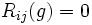$R_{ij}(g) = 0$ at all points.

## Metaproperties

### Direct product-closedness

This property of a Riemannian metric on a differential manifold is closed under taking direct products of manifolds

Given two Riemannian manifolds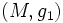$(M,g_1)$ and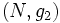$(N,g_2)$, such that both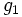$g_1$ and$g_2$ are Ricci-flat, the natural induced metric on$M \times N$ is also Ricci-flat.

## Flows

### Ricci flow

The Riemannian metrics with this property are precisely the stationary points for this flow: Ricci flow

The Ricci-flat metrics are precisely those metrics that are invariant under the Ricci flow. Ricci flows are intended to address the question: given a differential manifold, can we give it a Ricci-flat metric? The approach is to start with an arbitrary Riemannian metric, and then evolve it using the Ricci flow and take the limit as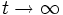$t \to \infty$.

Closely related are the notions of volume-normalized Ricci flow and Yamabe flow.